# Half derivative of x

The derivative of x is easy, it’s simply 1; the second derivative of x is the derivative of 1, which is 0; but what on earth is the half derivative of x??

When I came across this craziness, I was honestly shocked. I’d never thought of anything like that before, so I just couldn’t wrap my head around it. But don’t worry, it’s not as bad as it might seem.

Let’s start by giving a look at the result of first, second, third, fourth derivative of x to the first, second, third and fourth power to see if we can find any pattern: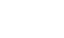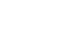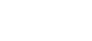There actually is a pattern! Take a look at this: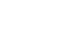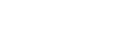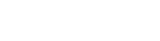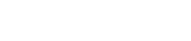This means the fifth derivative of x^5 is 5 · 4 · 3 · 2 · 1 = 120 and so on. This operation consisting of multiplying all numbers from n to 1 is called factorial and is represented by the symbol ‘!’.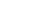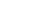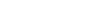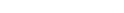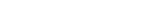Therefore,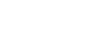In our case, however, we have the half derivative of x, i.e.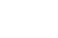Let’s do some examples: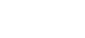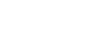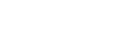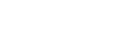From this we know for sure that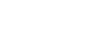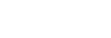The exponent of the x is equal to the order of the derivative. Now we have to figure out what k is.

Let’s decompose the coefficients of the x to see if there’s any pattern: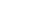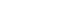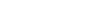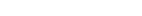As you can see, the exponent of the 2 is equal to the order n of the derivative, therefore we have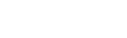Now we need to find j.

Notice that the greatest number in each multiplication has to do with n; 1680 is the coefficient of x^4 and, as we’ve just seen, the greatest term is 7, which is given by 4+3, where 4 is n. The same is for 120, where the highest term is 5, i.e. 3+2, where n is 3, and so on. Every time we sum n to n-1, so we know that the greatest term is equal to n+n-1=2n-1. We can also see that before 7 is 5, which is 7-2, or 2n-1-2, so 2n-3, then we have 3, which is 2n-5, ans as last is 1, i.e. 2n-7.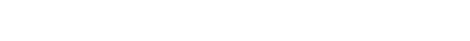1 · 3 · 5 · 7 looks a lot like 1 · 2 · 3 · 4 · 5 · 6 · 7, which is 7!; the only difference is that it’s all divided by 2 · 4 · 6: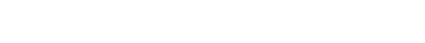Therefore,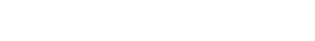We get the correct result! At this point we notice that 3 is equal to n-1 and 7 is equal to 2n-1, so we can generalize this expression for any n: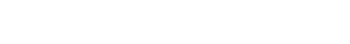This is the formula for the n-th derivative of x to the 2n: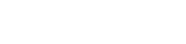Now we can find the half derivative of x: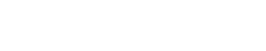The factorial function is limited to positive integers, such as 0, 1, 2, 3 and so on. For negative and/or non-integer numbers, the gamma function is used, which is actually the definition of (n-1)! using integrals, but I will talk about that in another post. To find the factorial of -1/2, just type (-1/2)! on Google or on a scientific calculator and this amazing result will show up: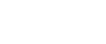Finally, we find that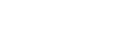That’s it!

I really hope you found this post interesting! If you liked it, give it a like, and subscribe for more! It would mean a lot to me!

Join 31 other subscribers.